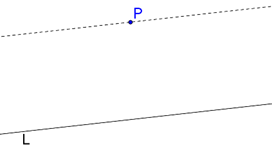# Euclid’s Axioms and Postulates

Euclid’s Axioms and Postulates

Euclid made use of the following axioms in his Elements. As you read these, take a moment to reflect on each axiom:

1. Things which are equal to the same thing are also equal to one another.

2. If equals be added to equals, the wholes are equal.

3. If equals be subtracted from equals, the remainders are equal.

4. Things which coincide with one another are equal to one another. For example, if two line segments AB and CD can be made to coincide with each other exactly, then we can say that they are equal, in the sense that they have equal lengths.

5. The whole is greater than the part.

6. Things which are double of the same things are equal to one another.

7. Things which are halves of the same things are equal to one another.

As you would have noticed, these axioms are general truths which would apply not only to geometry but to Mathematics in general. Next, let us take a look at the postulates of Euclid, which were (according to him) universal truths specific to geometry.

1.  First Postulate: A straight line may be drawn from any one point to any other. This postulate can be extended to say that a unique (one and only one) straight line may be drawn between any two points.

2. Second Postulate: A terminated line (a line segment) can be produced indefinitely.

3. Third Postulate: A circle can be drawn with any center and any radius.

4. Fourth Postulate: All right angles are equal to one another.

5. Fifth Postulate: Given a line L and a point P not on the line, exactly one line can be drawn through P which is parallel to L:The statement of the fifth postulate presented here is different from Euclid’s original version (more on this later).

Geometry
grade 9 | Questions Set 1
Geometry
Geometry
grade 9 | Questions Set 2
Geometry
More Important Topics
Numbers
Algebra
Geometry
Measurement
Money
Data
Trigonometry
Calculus
More Important Topics
Numbers
Algebra
Geometry
Measurement
Money
Data
Trigonometry
Calculus